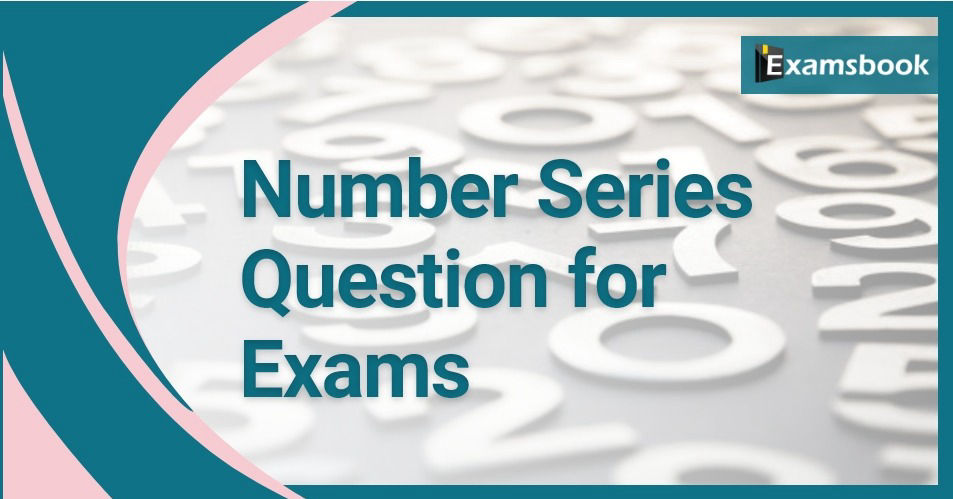• Save

# Number Series Questions for examsAll most in all the entrance tests, as well as interviews Number Series Questions, are asked to evaluate IQ. Solve these Number Series problems and practice for competitive exams. People who love mathematics definitely enjoy solving Number Series Questions and answers. This section is devoted to all such enthusiast brain buddies.

Let's have some important Number Series Questions and Answers to solve yourself for better practice of competitive exams. As well as of these questions, you should know Number Series Problems with Solutions for saving time in the competitive Exams.

## Number Series Questions and Answers logical reasoning problems:

Q :

127, 131, 139, ?, 151, 157, 163, 167,

(A) 149

(B) 147

(C) 141

(D) 143

Q :

2, 12, 36, 80, 150, ?

(A) 250

(B) 252

(C) 200

(D) 270

Explanation :

Explanation:

A unique pattern has been made into use in this series.

All numbers are (n^3 + n^2) where n is 1, 2, 3 and so on.

Q :

6, 14, 36, 98, ?

(A) 276

(B) 275

(C) 220

(D) 274

Explanation :

Explanation:
6 = 1^1 + 2^1 + 3^1
14 = 1^2 + 2^2 + 3^2
36 = 1^3 + 2^3 + 3^3
98 = 1^4 + 2^4 + 3^4
Thus the next number will be
1^5 + 2^5 + 3^5 = 276

Q :

5, 16, 49, 104, ?

(A) 171

(B) 191

(C) 181

(D) 161

Explanation :

Explanation:
+11 =16
+33 =49
+55 =104
+77 =181

Q :

8, 7, 11, 12, 14, 17, 17, 22, ?

(A) 27

(B) 20

(C) 24

(D) 22

Explanation :

Explanation:
There are two series (8, 11, 14, 17, 20) and (7, 12, 17, 22) increasing by 3 and 5 respectively.

Q :

2, 6, 12, 20, 30, 42, 56, ?

(A) 61

(B) 64

(C) 70

(D) 72

Explanation :

The pattern is 1 x 2, 2 x 3, 3 x 4, 4 x 5, 5 x 6, 6 x 7, 7 x 8.
So, the next number is 8 x 9 = 72.

Q :

4, -8, 16, -32, 64, ?

(A) 128

(B) -128

(C) 192

(D) -192

Explanation :

Explanation:
Each number is the proceeding number multiplied by -2.
So, the required number is -128.

Q :

7, 26, 63, 124, 215, 342, ?

(A) 481

(B) 511

(C) 391

(D) 421

Explanation :

Explanation:
Numbers are (23 – 1), (33 – 1), (43 – 1), (53 – 1), (63 – 1), (73 – 1) etc.
So, the next number is (83 – 1) = (512 – 1) = 511.

Q :

1, 6, 13, 22, 33, ?

(A) 44

(B) 45

(C) 46

(D) 47

Explanation :

The pattern is + 5, + 7, + 9, + 11,...
.'. Missing number = 33 + 13 = 46.[/

Q :

24, 60, 120, 210, ?

(A) 300

(B) 420

(C) 336

(D) 400